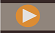## Statistics for Block Storage Applications

• General—General statistical information:

• Database start time—Start time according to the time zone of the database server

• Database elapsed time—Elapsed time in hours:minutes:seconds

• Number of connections—Number of connected users

• Blocks—Statistics about the data block of a block storage database:

• Number of existing blocks—Total number of blocks that exist (contain data)

• Block size—Size, in bytes, of each expanded (decompressed) data block (number of cells * 8; ideally, between 8 and 100 kilobytes). To alter block size, you must change the dense-sparse configuration of the database.

• Potential number of blocks—Maximum number of blocks (derived by multiplying the number of members of one sparse dimension by the number of members of another sparse dimension). For example, the Sample Basic database contains 19 Product members and 25 Market members (not counting shared or label-only members). Because Product and Market are sparse dimensions that store data, there are 19 x 25 = 475 potential data blocks.

• Existing level 0 blocks—Total number of level 0 blocks (blocks whose sparse dimension members have no children) that exist (contain data). Because data can be loaded at upper levels, level 0 blocks and blocks that are created by data input are not necessarily the same.

• Existing upper-level blocks—Total number of non-level 0 blocks that exist (contain data). Upper-level blocks include all combinations of upper-level sparse members plus upper-level combinations that include level 0 sparse members.

• Block density (%)—Average percentage fill of data points within each data block, based on a sample of existing data blocks. Dense-sparse configuration should maximize block density. Maximizing block density, however, may result in proliferation of data blocks. Block size and block proliferation considerations may overshadow the attempt to maximize block density.

• Percentage of maximum blocks existing—Percentage that compares the number of blocks that exist and the number of potential blocks. The percentage is a measure of the sparsity of the database. It is not uncommon for the percentage to be very small; for example, less than one percent.

• Compression ratio—Measure of the compression efficiency of blocks stored on disk. The compression ratio usually indicates block density.

• Average clustering ratio—Fragmentation level of data (.pag) files. The maximum value, 1, indicates no fragmentation. If you are experiencing degraded retrieval, calculation, or data load performance and the clustering ratio value is significantly less than 1, consider forcing a rewrite of data files by exporting and reloading data. Rewriting files defragments the files, resulting in a clustering ratio closer to 1.

• Average fragmentation quotient—Free space in a database. For example, an average fragmentation quotient value of 3.174765 means the database is 3% fragmented with free space. As you update and calculate data, empty spaces occur when a block can no longer fit in its original space and will either append at the end of the file or fit in another empty space that is large enough. The higher the number, the more empty spaces you have; therefore,the longer it takes to get to a particular record. The average fragmentation quotient helps you to decide if a restructure should be performed.

• Run Time

• Index files—Total number of index files.

• Page files—Total number of page files.

An index (.ind) or page (.pag) file reaches a maximum of 2 GB before another one is created. The number of index and page files shows an approximate database size to help troubleshoot performance issues. For example, if you have one index or page file, then the database size is greater than or equal to 2 GB. If you have two index or page files, then the database size is greater than or equal to 4 GB.

Tutorial Video:Managing BSO Database Properties